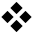Bootstrap Korean Grammar

Learn Korean Grammar step-by-step

# Korean grammar - Telling the time in Korean## Telling the time in Korean

The hour of the day (o'clock) is expressed using a native Korean numbers + .

-- While native Korean number one (1) is 하나, when using it to count objects it changes to ; So for one o'clock we say 한시. Likewise for the numbers 2, 3, and 4:

-- 두시, 세시, 네시 and therefore 열하나 열한시 and 열둘 열두시.

The minute of the hour is expressed using a Sino-Korean number +

Half past the hour is simply which means 'half'

오전 means AM and 오후 means PM.

정오 means 'noon' and 자정 means 'midnight'

Note that normally in written Korean the numbers are written in numeral form but in these examples they are spelled out to aid pronunciation.

 Examples: 지금 몇 시예요? What time is it?Literally: ?몇 means 'how many' or 'which' 한시예요. It is 1 o'clock.하나 (PK number) means 'one'하나 ⇒ 한시 두시 오분이에요. It is 5 past 2.다섯 (PK number) means 'five';둘 ⇒ 두시오 (SK number) means 'five' 세시 십분이에요. It is 10 past 3.셋 (PK number) means 'three'셋 ⇒ 세시십 (SK number) means 'ten' 오후 네시 십오분이에요. It is 15 past 4 PM.넷 (PK number) means 'four'넷 ⇒ 네시십오 (SK number) means 'fiften' 지금 다섯시 이십이분이에요. It is 22 past 5 now.다섯 (PK number) means 'five'이십이 (SK number) means 'twenty two' 도쿄는 여섯시 반이에요. It is half past 6 in Tokyo.여섯 (PK number) means 'six'반 means 'half' 뉴욕은 오전 일곱시 사십오분이에요. It is 7:45 AM in New York.일곱 (PK number) means 'seven'사십오 (SK number) means 'forty five' 여덟시 오십구분이에요. It is 8:59.여덟 (PK number) means 'eight'오십구 (SK number) means 'fifty nine' 아홉시는 너무 늦었다. 9 o'clock was too late.아홉 (PK number) means 'nine' 열시 사십오분은 너무 이른 시간입니다. 10:45 is very early.열 (PK number) means 'ten'사십오 (SK number) means 'forty five' 아직 열한시예요? Is it 11 o'clock yet?열하나 (PK number) means 'eleven'열하나 ⇒ 열한시 비행기는 몇 시에 도착해요? At what time does the plane arrive?몇 시에 means 'at what time' 비행기는 정오에 도착해요. The plane arrives at noon.정오 means 'noon' 정오는 오후 열두시예요. Noon is at 12 PM.오후 means 'PM' 나는 자정에 잠자리에 들어요. I go to bed at midnight.자정 means 'midnight' 자정은 오전 열두시예요. Midnight is 12 AM.열둘 (PK number) means 'twelve'열둘 ⇒ 열두시